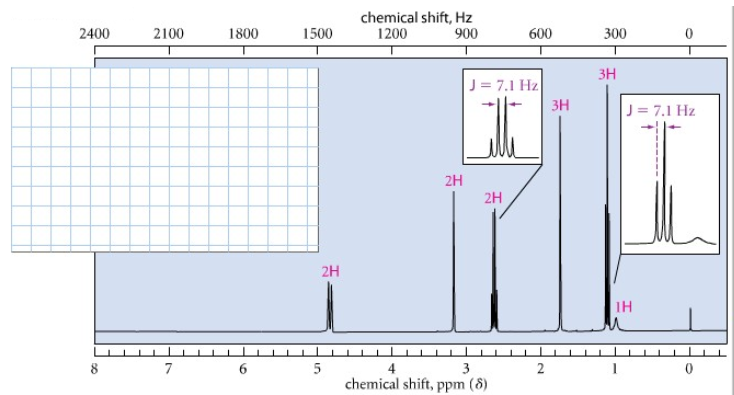# Problem: Provide a structure for the following compound:C6H13N; IR: 3280, 1653, 898 cm^-1;1H NMR spectrum:

###### FREE Expert Solution

Given molecular formula: C6H13N

IHD:

$\overline{){\mathbf{IHD}}{\mathbf{=}}\frac{\mathbf{\left[}\mathbf{2}\mathbf{n}\mathbf{+}\mathbf{2}\mathbf{\right]}\mathbf{-}\mathbf{H}}{\mathbf{2}}}$

where n = number of carbons and H is:

+1 for H or X

0 for O

• —1 for N

87% (44 ratings)###### Problem Details

Provide a structure for the following compound:

C6H13N;

IR: 3280, 1653, 898 cm^-1;

1H NMR spectrum: